This document contains the power analysis for the primary models of interest. For each model, we display the power to detect significant $$\tau$$ values, or different standard deviations of between-study effects (reported in the metric of odds ratios). We also mark the level of $$tau$$ estimated by our model and the power to detect this effect. We note here that we are well powered to detect between-study variability that is commonly found in psychology (van Erp, Verhagen, Grasman, & Wagenmakers, 2017).

Many thanks to Brenton Wiernik, who developed the code to calculate power based on our analyses!

The following packages were used to generate this figure:

# Cross-sectional

## Hypertension/high blood pressure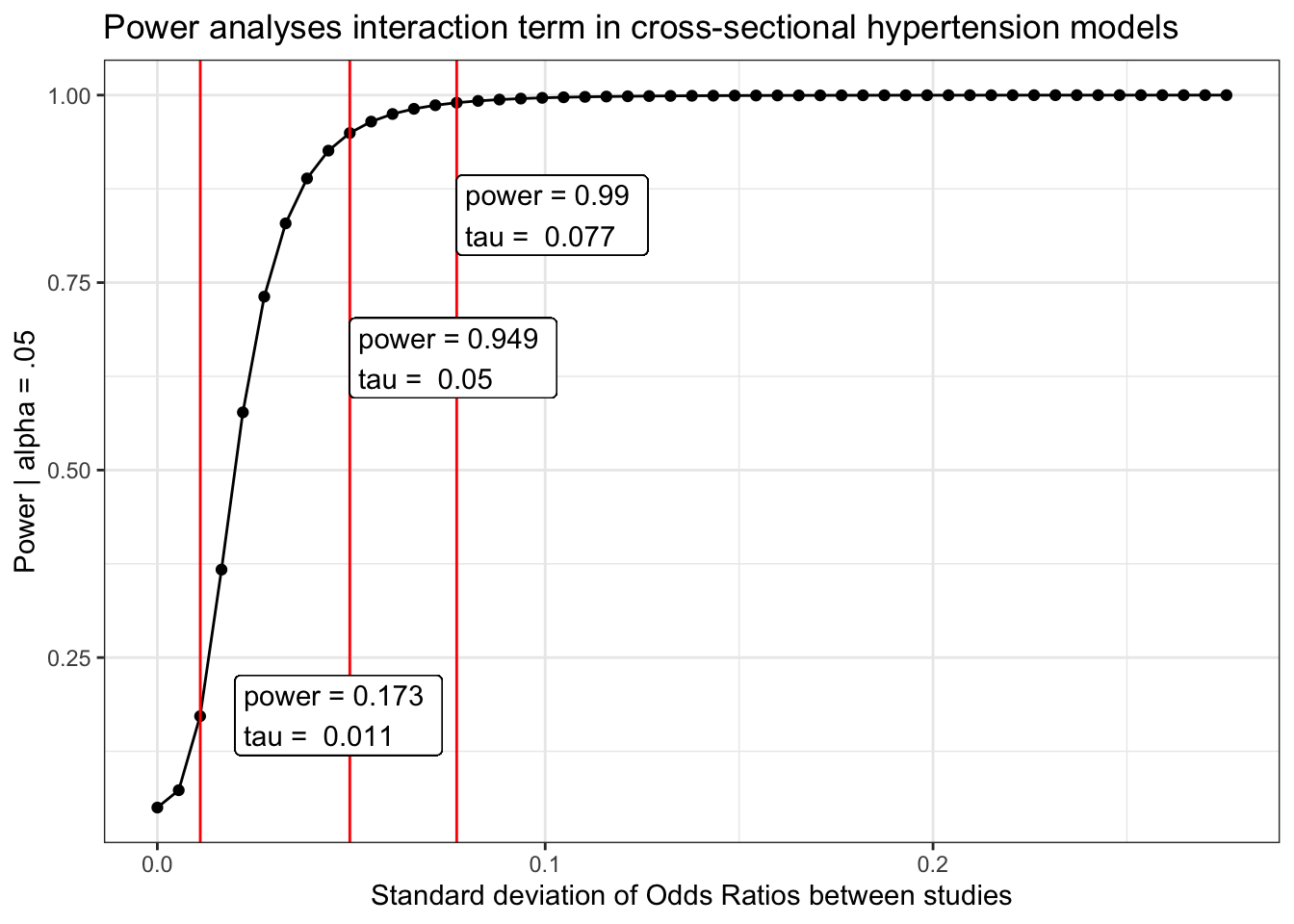## Diabetes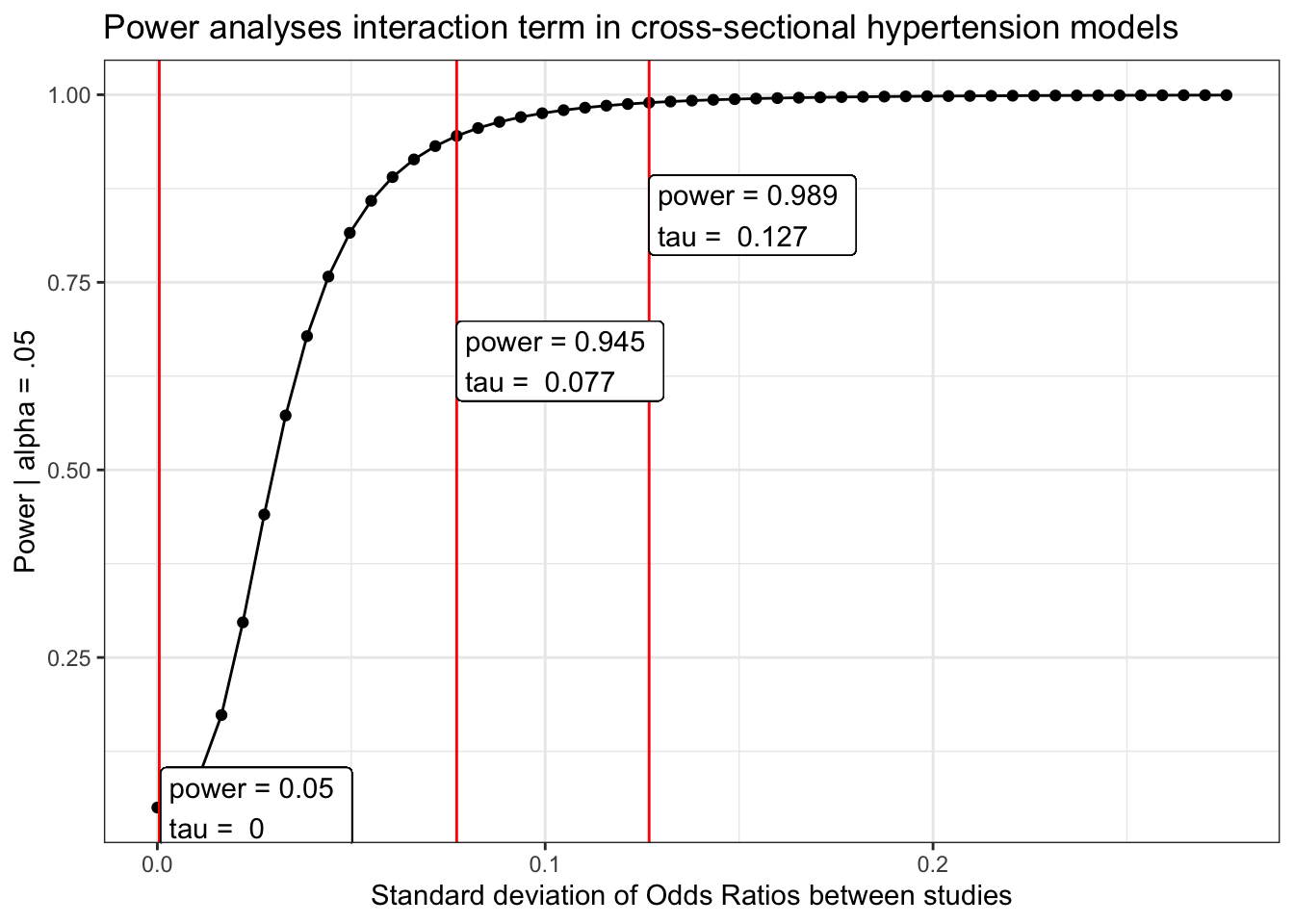## Heart disease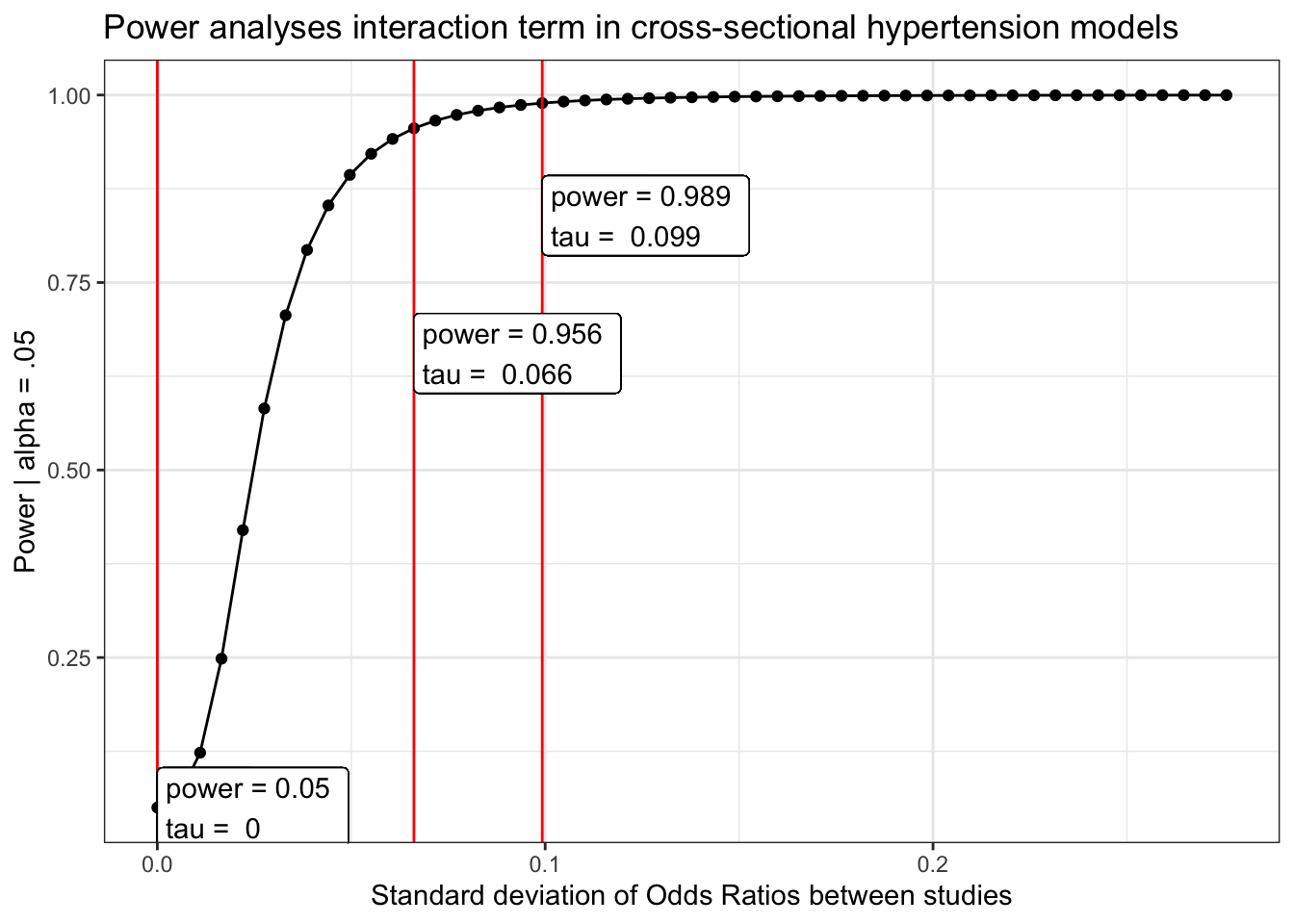# Longitudinal

## Hypertension/high blood pressure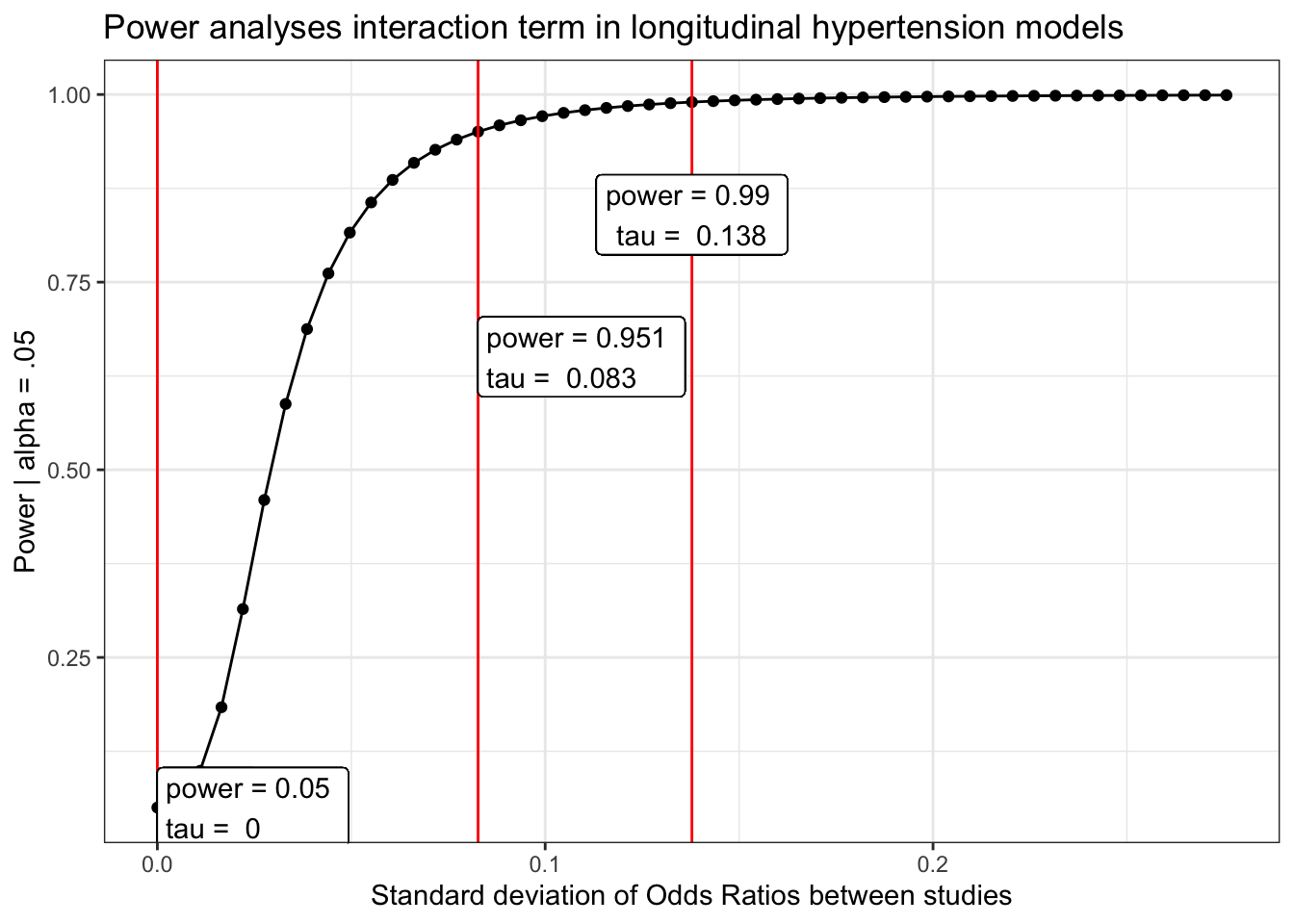## Diabetes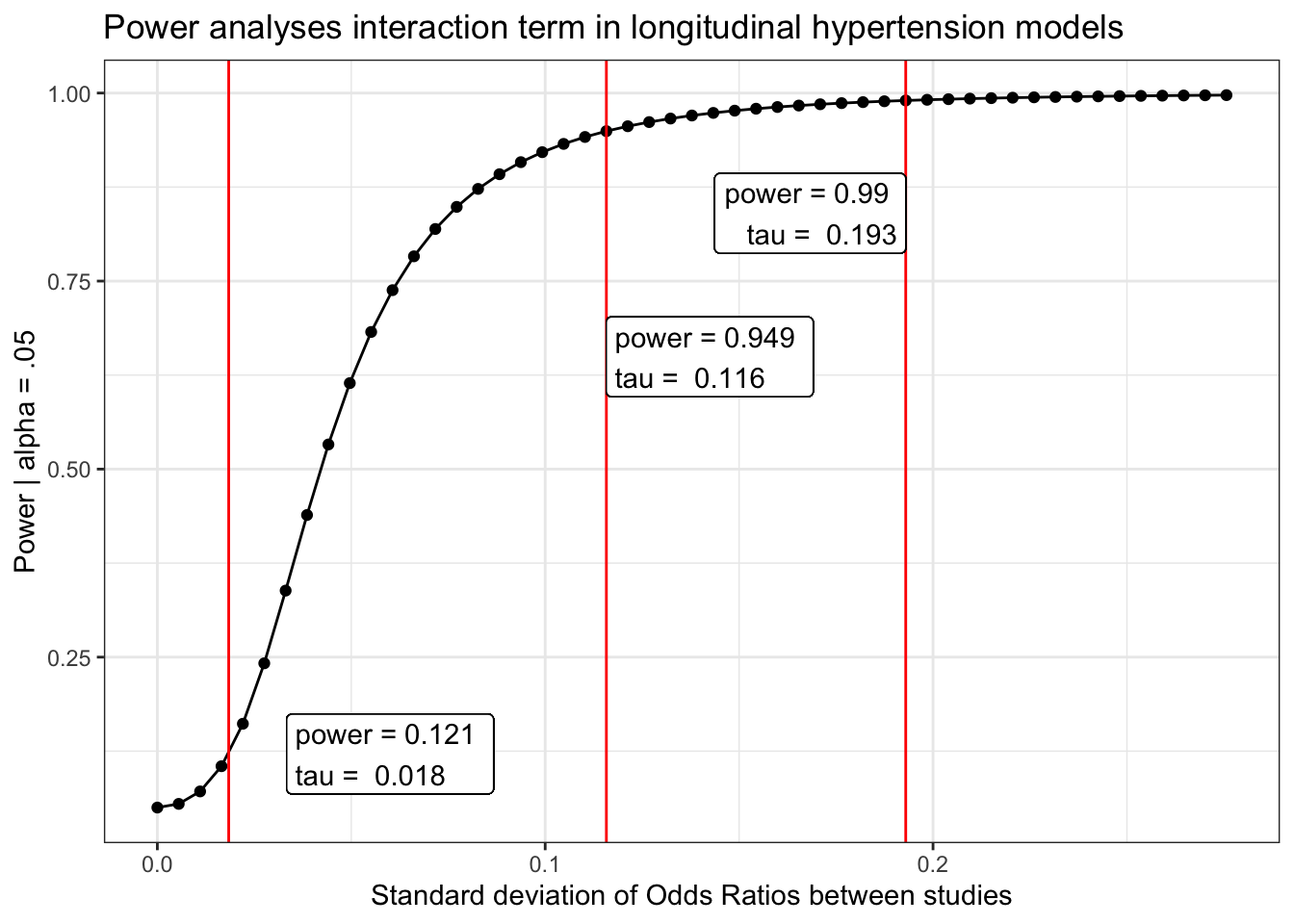## Heart disease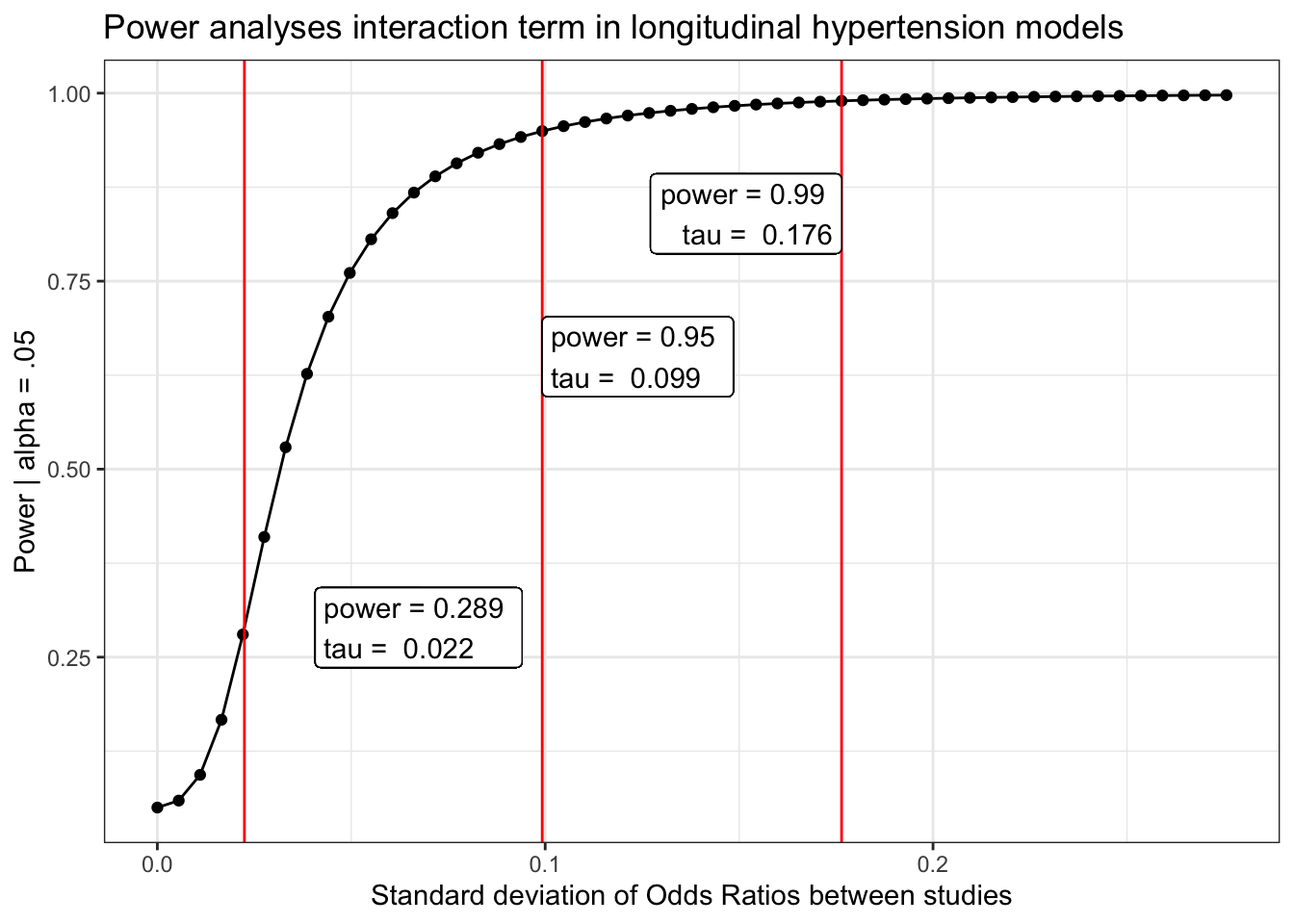# Code

# Based on methods described by Hedges and Pigott, https://doi.org/10.1037/1082-989X.9.4.426, p. 438
# Written 2019-10-25 by Brenton M. Wiernik

power_curve <- function(object, tau, level = .95) {
if (! inherits(object, "rma.uni")) stop("'object' must be an object of class 'rma.uni'")
tau2 <- tau^2
obs_tau2 <- object$tau2 obs_tau <- sqrt(obs_tau2) obs_H2 <- object$H2
s2 <- obs_tau2 / (obs_H2 - 1)
df <- object$k - object$p
crit <- qchisq(.95, df)
wi <- 1 / object$vi a <- sum(wi) - sum(wi^2) / sum(wi) mu_q <- function(tau2) a * tau2 + df b <- function(tau2) df + 2 * a * tau2 + (sum(wi^2) - 2 * sum(wi^3) / sum(wi) + sum(wi^2)^2 / sum(wi)^2) * tau2^2 var_q <- function(tau2) 2 * b(tau2) r <- function(tau2) var_q(tau2) / (2 * mu_q(tau2)) s <- function(tau2) 2 * mu_q(tau2)^2 / var_q(tau2) H <- function(tau2) pchisq(crit / r(tau2), s(tau2)) power <- 1 - H(tau2) out <- list(obs_tau = obs_tau, power = data.frame(tau = tau, power = power)) return(out) } The following packages were used to generate this figure: library(tidyverse) library(metafor) library(papaja) library(here) tau = seq(0, .5, .01) # possible values of the standard deviation of effect sizes ## Cross-sectional ### Hypertension/high blood pressure load(here("chronic/meta output/hbp_cross.Rdata")) power_hbp_cross = power_curve(meta.int.mod3, tau, level = .95) obs = power_curve(meta.int.mod3, power_hbp_cross$obs_tau, level =.95)$power[1,2] #for which tau are we powered? pwr.95=.95 pwr.99=.99 tau.95 = which(abs(power_hbp_cross$power$power-pwr.95)==min(abs(power_hbp_cross$power$power-pwr.95))) pwr.95 = power_hbp_cross$power$power[tau.95] tau.95 = power_hbp_cross$power$tau[tau.95] tau.99 = which(abs(power_hbp_cross$power$power-pwr.99)==min(abs(power_hbp_cross$power$power-pwr.99))) pwr.99 = power_hbp_cross$power$power[tau.99] tau.99 = power_hbp_cross$power$tau[tau.99] power_hbp_cross$power %>%
mutate(tau_d = tau/(pi/sqrt(3))) %>%
ggplot(aes(x = tau_d, y = power)) +
geom_point()+
geom_line()+
geom_vline(aes(xintercept = power_hbp_cross$obs_tau/(pi/sqrt(3))), color = "red") + geom_vline(aes(xintercept = tau.95/(pi/sqrt(3))), color = "red") + geom_vline(aes(xintercept = tau.99/(pi/sqrt(3))), color = "red") + geom_label(aes(x = power_hbp_cross$obs_tau, y = obs,
label = paste("power =", round(obs, 3),
"\ntau = ", round( power_hbp_cross$obs_tau/(pi/sqrt(3)), 3))), hjust = "inward")+ geom_label(aes(x = tau.95/(pi/sqrt(3)), y = pwr.95-.3, label = paste("power =", round(pwr.95, 3), "\ntau = ", round( tau.95/(pi/sqrt(3)), 3))), hjust = "inward")+ geom_label(aes(x = tau.99/(pi/sqrt(3)), y = pwr.99-.15, label = paste("power =", round(pwr.99, 3), "\ntau = ", round( tau.99/(pi/sqrt(3)), 3))), hjust = "inward")+ labs(x = "Standard deviation of Odds Ratios between studies", y = "Power | alpha = .05", title = "Power analyses interaction term in cross-sectional hypertension models") + theme_bw() ### Diabetes load(here("chronic/meta output/diabetes_cross.Rdata")) power_diabetes_cross = power_curve(meta.int.mod3, tau, level = .95) obs = power_curve(meta.int.mod3, power_diabetes_cross$obs_tau, level =.95)$power[1,2] #for which tau are we powered? pwr.95=.95 pwr.99=.99 tau.95 = which(abs(power_diabetes_cross$power$power-pwr.95)==min(abs(power_diabetes_cross$power$power-pwr.95))) pwr.95 = power_diabetes_cross$power$power[tau.95] tau.95 = power_diabetes_cross$power$tau[tau.95] tau.99 = which(abs(power_diabetes_cross$power$power-pwr.99)==min(abs(power_diabetes_cross$power$power-pwr.99))) pwr.99 = power_diabetes_cross$power$power[tau.99] tau.99 = power_diabetes_cross$power$tau[tau.99] power_diabetes_cross$power %>%
mutate(tau_d = tau/(pi/sqrt(3))) %>%
ggplot(aes(x = tau_d, y = power)) +
geom_point()+
geom_line()+
geom_vline(aes(xintercept = power_diabetes_cross$obs_tau/(pi/sqrt(3))), color = "red") + geom_vline(aes(xintercept = tau.95/(pi/sqrt(3))), color = "red") + geom_vline(aes(xintercept = tau.99/(pi/sqrt(3))), color = "red") + geom_label(aes(x = power_diabetes_cross$obs_tau, y = obs,
label = paste("power =", round(obs, 3),
"\ntau = ", round( power_diabetes_cross$obs_tau/(pi/sqrt(3)), 3))), hjust = "inward")+ geom_label(aes(x = tau.95/(pi/sqrt(3)), y = pwr.95-.3, label = paste("power =", round(pwr.95, 3), "\ntau = ", round( tau.95/(pi/sqrt(3)), 3))), hjust = "inward")+ geom_label(aes(x = tau.99/(pi/sqrt(3)), y = pwr.99-.15, label = paste("power =", round(pwr.99, 3), "\ntau = ", round( tau.99/(pi/sqrt(3)), 3))), hjust = "inward")+ labs(x = "Standard deviation of Odds Ratios between studies", y = "Power | alpha = .05", title = "Power analyses interaction term in cross-sectional hypertension models") + theme_bw() ### Heart disease load(here("chronic/meta output/heart_cross.Rdata")) power_heart_cross = power_curve(meta.int.mod3, tau, level = .95) obs = power_curve(meta.int.mod3, power_heart_cross$obs_tau, level =.95)$power[1,2] #for which tau are we powered? pwr.95=.95 pwr.99=.99 tau.95 = which(abs(power_heart_cross$power$power-pwr.95)==min(abs(power_heart_cross$power$power-pwr.95))) pwr.95 = power_heart_cross$power$power[tau.95] tau.95 = power_heart_cross$power$tau[tau.95] tau.99 = which(abs(power_heart_cross$power$power-pwr.99)==min(abs(power_heart_cross$power$power-pwr.99))) pwr.99 = power_heart_cross$power$power[tau.99] tau.99 = power_heart_cross$power$tau[tau.99] power_heart_cross$power %>%
mutate(tau_d = tau/(pi/sqrt(3))) %>%
ggplot(aes(x = tau_d, y = power)) +
geom_point()+
geom_line()+
geom_vline(aes(xintercept = power_heart_cross$obs_tau/(pi/sqrt(3))), color = "red") + geom_vline(aes(xintercept = tau.95/(pi/sqrt(3))), color = "red") + geom_vline(aes(xintercept = tau.99/(pi/sqrt(3))), color = "red") + geom_label(aes(x = power_heart_cross$obs_tau, y = obs,
label = paste("power =", round(obs, 3),
"\ntau = ", round( power_heart_cross$obs_tau/(pi/sqrt(3)), 3))), hjust = "inward")+ geom_label(aes(x = tau.95/(pi/sqrt(3)), y = pwr.95-.3, label = paste("power =", round(pwr.95, 3), "\ntau = ", round( tau.95/(pi/sqrt(3)), 3))), hjust = "inward")+ geom_label(aes(x = tau.99/(pi/sqrt(3)), y = pwr.99-.15, label = paste("power =", round(pwr.99, 3), "\ntau = ", round( tau.99/(pi/sqrt(3)), 3))), hjust = "inward")+ labs(x = "Standard deviation of Odds Ratios between studies", y = "Power | alpha = .05", title = "Power analyses interaction term in cross-sectional hypertension models") + theme_bw() ## Longitudinal ### Hypertension/high blood pressure load(here("chronic/meta output/hbp_long.Rdata")) power_hbp_long = power_curve(meta.int.mod3, tau, level = .95) obs = power_curve(meta.int.mod3, power_hbp_long$obs_tau, level =.95)$power[1,2] #for which tau are we powered? pwr.95=.95 pwr.99=.99 tau.95 = which(abs(power_hbp_long$power$power-pwr.95)==min(abs(power_hbp_long$power$power-pwr.95))) pwr.95 = power_hbp_long$power$power[tau.95] tau.95 = power_hbp_long$power$tau[tau.95] tau.99 = which(abs(power_hbp_long$power$power-pwr.99)==min(abs(power_hbp_long$power$power-pwr.99))) pwr.99 = power_hbp_long$power$power[tau.99] tau.99 = power_hbp_long$power$tau[tau.99] power_hbp_long$power %>%
mutate(tau_d = tau/(pi/sqrt(3))) %>%
ggplot(aes(x = tau_d, y = power)) +
geom_point()+
geom_line()+
geom_vline(aes(xintercept = power_hbp_long$obs_tau/(pi/sqrt(3))), color = "red") + geom_vline(aes(xintercept = tau.95/(pi/sqrt(3))), color = "red") + geom_vline(aes(xintercept = tau.99/(pi/sqrt(3))), color = "red") + geom_label(aes(x = power_hbp_long$obs_tau, y = obs,
label = paste("power =", round(obs, 3),
"\ntau = ", round( power_hbp_long$obs_tau/(pi/sqrt(3)), 3))), hjust = "inward")+ geom_label(aes(x = tau.95/(pi/sqrt(3)), y = pwr.95-.3, label = paste("power =", round(pwr.95, 3), "\ntau = ", round( tau.95/(pi/sqrt(3)), 3))), hjust = "inward")+ geom_label(aes(x = tau.99/(pi/sqrt(3)), y = pwr.99-.15, label = paste("power =", round(pwr.99, 3), "\ntau = ", round( tau.99/(pi/sqrt(3)), 3))), hjust = "inward")+ labs(x = "Standard deviation of Odds Ratios between studies", y = "Power | alpha = .05", title = "Power analyses interaction term in longitudinal hypertension models") + theme_bw() ### Diabetes load(here("chronic/meta output/diabetes_long.Rdata")) power_diabetes_long = power_curve(meta.int.mod3, tau, level = .95) obs = power_curve(meta.int.mod3, power_diabetes_long$obs_tau, level =.95)$power[1,2] #for which tau are we powered? pwr.95=.95 pwr.99=.99 tau.95 = which(abs(power_diabetes_long$power$power-pwr.95)==min(abs(power_diabetes_long$power$power-pwr.95))) pwr.95 = power_diabetes_long$power$power[tau.95] tau.95 = power_diabetes_long$power$tau[tau.95] tau.99 = which(abs(power_diabetes_long$power$power-pwr.99)==min(abs(power_diabetes_long$power$power-pwr.99))) pwr.99 = power_diabetes_long$power$power[tau.99] tau.99 = power_diabetes_long$power$tau[tau.99] power_diabetes_long$power %>%
mutate(tau_d = tau/(pi/sqrt(3))) %>%
ggplot(aes(x = tau_d, y = power)) +
geom_point()+
geom_line()+
geom_vline(aes(xintercept = power_diabetes_long$obs_tau/(pi/sqrt(3))), color = "red") + geom_vline(aes(xintercept = tau.95/(pi/sqrt(3))), color = "red") + geom_vline(aes(xintercept = tau.99/(pi/sqrt(3))), color = "red") + geom_label(aes(x = power_diabetes_long$obs_tau, y = obs,
label = paste("power =", round(obs, 3),
"\ntau = ", round( power_diabetes_long$obs_tau/(pi/sqrt(3)), 3))), hjust = "inward")+ geom_label(aes(x = tau.95/(pi/sqrt(3)), y = pwr.95-.3, label = paste("power =", round(pwr.95, 3), "\ntau = ", round( tau.95/(pi/sqrt(3)), 3))), hjust = "inward")+ geom_label(aes(x = tau.99/(pi/sqrt(3)), y = pwr.99-.15, label = paste("power =", round(pwr.99, 3), "\ntau = ", round( tau.99/(pi/sqrt(3)), 3))), hjust = "inward")+ labs(x = "Standard deviation of Odds Ratios between studies", y = "Power | alpha = .05", title = "Power analyses interaction term in longitudinal hypertension models") + theme_bw() ### Heart disease load(here("chronic/meta output/heart_long.Rdata")) power_heart_long = power_curve(meta.int.mod3, tau, level = .95) obs = power_curve(meta.int.mod3, power_heart_long$obs_tau, level =.95)$power[1,2] #for which tau are we powered? pwr.95=.95 pwr.99=.99 tau.95 = which(abs(power_heart_long$power$power-pwr.95)==min(abs(power_heart_long$power$power-pwr.95))) pwr.95 = power_heart_long$power$power[tau.95] tau.95 = power_heart_long$power$tau[tau.95] tau.99 = which(abs(power_heart_long$power$power-pwr.99)==min(abs(power_heart_long$power$power-pwr.99))) pwr.99 = power_heart_long$power$power[tau.99] tau.99 = power_heart_long$power$tau[tau.99] power_heart_long$power %>%
mutate(tau_d = tau/(pi/sqrt(3))) %>%
ggplot(aes(x = tau_d, y = power)) +
geom_point()+
geom_line()+
geom_vline(aes(xintercept = power_heart_long$obs_tau/(pi/sqrt(3))), color = "red") + geom_vline(aes(xintercept = tau.95/(pi/sqrt(3))), color = "red") + geom_vline(aes(xintercept = tau.99/(pi/sqrt(3))), color = "red") + geom_label(aes(x = power_heart_long$obs_tau, y = obs,
label = paste("power =", round(obs, 3),
"\ntau = ", round( power_heart_long\$obs_tau/(pi/sqrt(3)), 3))), hjust = "inward")+
geom_label(aes(x = tau.95/(pi/sqrt(3)), y = pwr.95-.3,
label = paste("power =", round(pwr.95, 3),
"\ntau = ", round( tau.95/(pi/sqrt(3)), 3))), hjust = "inward")+
geom_label(aes(x = tau.99/(pi/sqrt(3)), y = pwr.99-.15,
label = paste("power =", round(pwr.99, 3),
"\ntau = ", round( tau.99/(pi/sqrt(3)), 3))), hjust = "inward")+
labs(x = "Standard deviation of Odds Ratios between studies",
y = "Power | alpha = .05",
title = "Power analyses interaction term in longitudinal hypertension models") +
theme_bw()